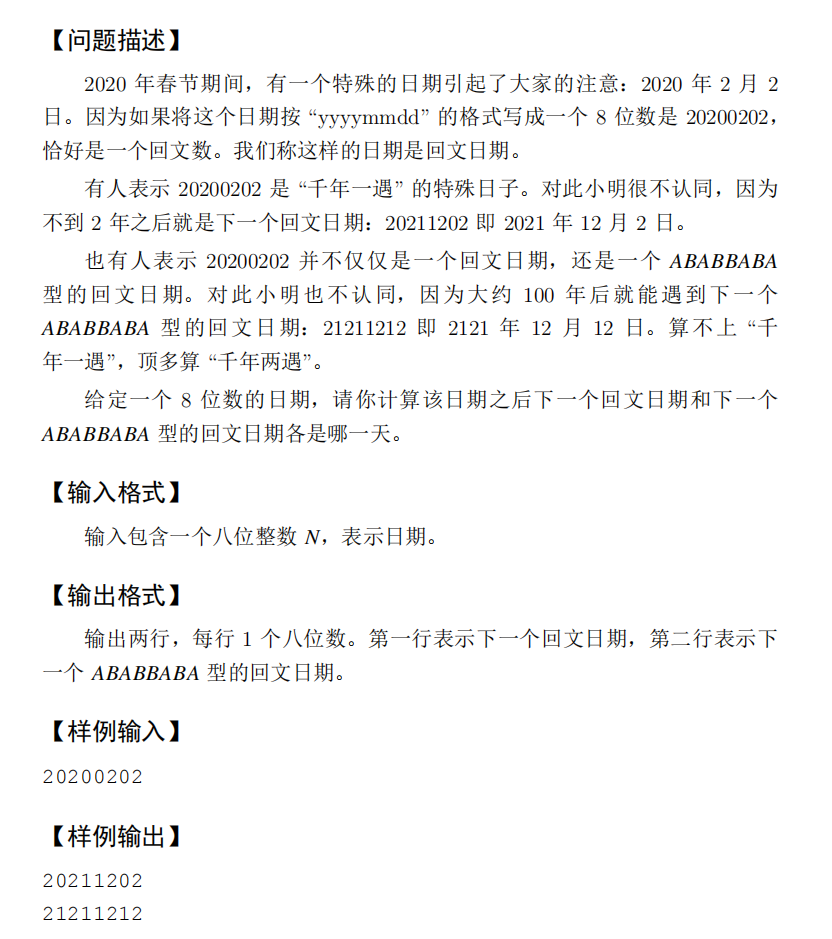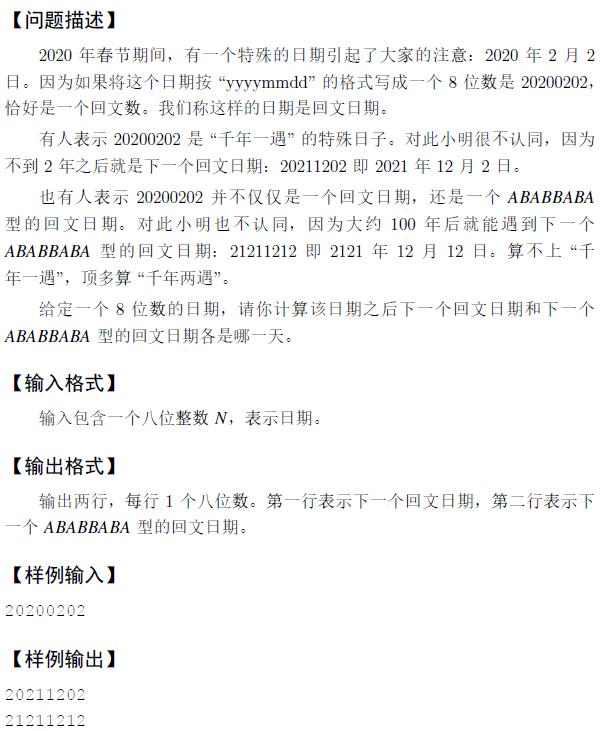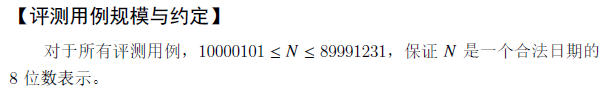• 蓝桥杯回文日期
2022-02-12 22:16:53

### 题目描述

2020 年春节期间，有一个特殊的日期引起了大家的注意：2020 年 2 月 2 日。因为如果将这个日期按 “yyyymmdd” 的格式写成一个 8 位数是 20200202，恰好是一个回文数。我们称这样的日期是回文日期。

有人表示 20200202 是 “千年一遇” 的特殊日子。对此小明很不认同，因为不到 2 年之后就是下一个回文日期：20211202 即 2021 年 12 月 2 日。

也有人表示 20200202 并不仅仅是一个回文日期，还是一个 ABABBABA 型的回文日期。对此小明也不认同，因为大约 100 年后就能遇到下一个 ABABBABA 型的回文日期：21211212 即 2121 年 12 月 12 日。算不上 “千年一遇”，顶多算 “千年两遇”。

给定一个 8 位数的日期，请你计算该日期之后下一个回文日期和下一个 ABABBABA 型的回文日期各是哪一天。

### 输入描述

输入包含一个八位整数 NN，表示日期。

对于所有评测用例，10000101 \leq N \leq 8999123110000101≤N≤89991231，保证 NN 是一个合法日期的 8 位数表示。

### 输出描述

输出两行，每行 1 个八位数。第一行表示下一个回文日期，第二行表示下一个 ABABBABA 型的回文日期。

### 输入输出样例

示例

输入

20200202


输出

20211202
21211212


### 运行限制

• 最大运行时间：1s
• 最大运行内存: 256M

## 解体思路：

先看下第一数20200202，我们先把这个数对半分开(如题目中提到的yyyymmdd一样，任何一个日期都是八位数，所以我们把它对半分开)，前半部分是2020，后面是0202，我们观察这两个数，明显的可以看到后面的0202就是reserve(2020)一下，所以下一个回文数就是把2020的后面的一个数即2021reserve一下，就是样例输出里面的20211202，同理20211202的下一个回文数就是把2021的后一个数reserve一下，即20222202

下面根据这一个思路写一下题

#include<iostream>
using namespace std;
const int N = 10000;
int month = { 0, 31, 28, 31, 30, 31, 30, 31, 31, 30, 31, 30, 31 };//先准备一个月份的数组
#define ll long long int
int main()
{
ll n;
ll f;//
ll ff;
cin>>n;//样例中输入的是一个八位数，根据刚才的思路，我们已经知道了下一个回文数只
//和前面的四位数有关，所以下面需要整除一下
int m = n / N;
//下面直接遍历吧
while(true)
{
int a = pan(m);//这里pan是我们准备的一个获得目标的函数(即reserves的部分）下面在说
//对了，当不符合条件时pan()函数返回的是-1
//下面判断一下时候要结束循环，当a!=-1时结束循环，因为a!=-1就代表着找到了目标
if(a!=-1)
{
f = m*N+a;
//下面还要判断一下f是不是大与n，因为当我们输入20200202时，我们找到的第一个回文数
//就是20200202，但是我们要找的它后面的
if(f>n) break;
m++;//没有找到继续循环
}
}

return 0;
}

在看下第二个输出样例，它的要求是ABABBABA型的 ，我们先把它分成两部分ABAB和BABA，我们会发现这里和上面提到的一样，但是前部分的ABAB还可以分成AB*100+AB，所以这个我们只需要管前面的两位数就行

#include<iostream>
using namespace std;
const int N = 10000;
int month = { 0, 31, 28, 31, 30, 31, 30, 31, 31, 30, 31, 30, 31 };//先准备一个月份的数组
#define ll long long int
int main()
{
ll n;
ll f;//
ll ff;
cin>>n;//样例中输入的是一个八位数，根据刚才的思路，我们已经知道了下一个回文数只
//和前面的四位数有关，所以下面需要整除一下
int m = n / N;
//下面直接遍历吧
while(true)
{
int a = pan(m);//这里pan是我们准备的一个获得目标的函数(即reserves的部分）下面在说
//对了，当不符合条件时pan()函数返回的是-1
//下面判断一下时候要结束循环，当a!=-1时结束循环，因为a!=-1就代表着找到了目标
if(a!=-1)
{
f = m*N+a;
//下面还要判断一下f是不是大与n，因为当我们输入20200202时，我们找到的第一个回文数
//就是20200202，但是我们要找的它后面的
if(max>n) break;
m++;//没有找到继续循环
}
}
//直接从这看
int b = n/N/100;
while(true)
{
if(b/10 == b%10)continue;//会遇到11这样的不符合AB类型的，所以判断一下，不符合的直接跳
//过
int c = b*100+b;//给它能成四位数,让它也能使用pan()函数

int a = pan(c);
if(a!=-1)
{
ff = c*N+a;
if(ff>n)break;
}
b++;
}
cout<<f<<endl<<ff;
return 0;
}

下面在看下pan()函数

ll pan(int n)
{
int a = n % 10 * 1000;
int b = n / 10 % 10 * 100;
int c = n / 100 % 10 * 10;
int d = n / 1000;
int m = a + b + c + d;
if (m / 100 > 12) return -1;//先判断下月份是否小于等于12，大于的直接返回-1
if (n % 400 == 0 || (n % 4 == 0 && n % 100 != 0))//判断想是否是闰年
{
if (m / 100 == 2)//判断想是否是2月
{
if (m % 100 <= month[m / 100] + 1)//因为我们month里面存的是28，所以需要+1
return m;
}
else
{
if (m % 100 <= month[m / 100])
return m;
}
}
if (m % 100 <= month[m / 100])
return m;
else return -1;
}

总代码

#include<iostream>
using namespace std;
const int N = 10000;
int month = { 0, 31, 28, 31, 30, 31, 30, 31, 31, 30, 31, 30, 31 };//先准备一个月份的数组
#define ll long long int
ll pan(int n)
{
int a = n % 10 * 1000;
int b = n / 10 % 10 * 100;
int c = n / 100 % 10 * 10;
int d = n / 1000;
int m = a + b + c + d;
if (m / 100 > 12) return -1;//先判断下月份是否小于等于12，大于的直接返回-1
if (n % 400 == 0 || (n % 4 == 0 && n % 100 != 0))//判断想是否是闰年
{
if (m / 100 == 2)//判断想是否是2月
{
if (m % 100 <= month[m / 100] + 1)//因为我们month里面存的是28，所以需要+1
return m;
}
else
{
if (m % 100 <= month[m / 100])
return m;
}
}
if (m % 100 <= month[m / 100])
return m;
else return -1;
}
int main()
{
ll n;
ll f;//
ll ff;
cin>>n;//样例中输入的是一个八位数，根据刚才的思路，我们已经知道了下一个回文数只
//和前面的四位数有关，所以下面需要整除一下
int m = n / N;
//下面直接遍历吧
while(true)
{
int a = pan(m);//这里pan是我们准备的一个获得目标的函数(即reserves的部分）下面在说
//对了，当不符合条件时pan()函数返回的是-1
//下面判断一下时候要结束循环，当a!=-1时结束循环，因为a!=-1就代表着找到了目标
if(a!=-1)
{
f = m*N+a;
//下面还要判断一下f是不是大与n，因为当我们输入20200202时，我们找到的第一个回文数
//就是20200202，但是我们要找的它后面的
if(f>n) break;
m++;//没有找到继续循环
}
}
//直接从这看
int b = n/N/100;
while(true)
{
if(b/10 == b%10)continue;//会遇到11这样的不符合AB类型的，所以判断一下，不符合的直接跳
//过
int c = b*100+b;//给它能成四位数,让它也能使用pan()函数

int a = pan(c);
if(a!=-1)
{
ff = c*N+a;
if(ff>n)break;
}
b++;
}
cout<<f<<endl<<ff;
return 0;
}

上面这个写的有点问题，但是思路每错

下面这个代码能通过

#include<iostream>
using namespace std;
#define ll long long int
const int N = 10000;
int month = { 0, 31, 28, 31, 30, 31, 30, 31, 31, 30, 31, 30, 31 };
ll pan(int n)
{
int a = n % 10 * 1000;
int b = n / 10 % 10 * 100;
int c = n / 100 % 10 * 10;
int d = n / 1000;
int m = a + b + c + d;
if (m / 100 > 12) return -1;
if (n % 400 == 0 || (n % 4 == 0 && n % 100 != 0))
{
if (m / 100 == 2)
{
if (m % 100 <= month[m / 100] + 1)
return m;
}
else
{
if (m % 100 <= month[m / 100])
return m;
}
}
if (m % 100 <= month[m / 100])
return m;
else return -1;
}
int main()
{
ll n;
ll max;
ll min;
cin >> n;
int m = n / N;
while (true)
{
int a = pan(m);
if (a != -1)
{
max = m * N + a;
if (max > n) break;
}
m++;
}
int b = (n / N / 100);
while (true)
{
int c = b * 100 + b;
int a = pan(c);
if (a != -1)
{
min = c * N + a;
if (min > n && min%10 !=min%100/10)break;
}
b++;
}
cout << max << endl << min;
return 0;
}

更多相关内容
• 这道题好像是一个蓝桥杯的省赛题，但是具体的是哪一个省的，我也不不记得了，也不想...我们称这样的日期是回文日期。有人表示 20200202 是“千年一遇” 的特殊日子。对此小明很不认同，因为不到 2 年之后就是下一个回文

## 这道题好像是一个蓝桥杯的省赛题，但是具体的是哪一个省的，我也不不记得了，也不想去搜了，反正今天就把它写到这里，有需要的博客主可以自行观看，好了，废话不多说，直接上题。

### 题目描述：

##### 注意

下一个回文日期和下一个 ABABBABA 型的回文日期可能是同一天。

ABABBABA 型的回文日期，需要满足 A≠B。

#### 输入格式

输入包含一个八位整数 N，表示日期。

### 输出格式

输出两行，每行 1 个八位数。

第一行表示下一个回文日期，第二行表示下一个 ABABBABA 型的回文日期。

###### 数据范围

对于所有评测用例，10000101≤N≤89991231，保证 N 是一个合法日期的 8 位数表示。

20200202

20211202
21211212

###### 初看这道题感觉代码逻辑还是比较简单的，当然这个题难度测试本来就是简单的，但是对于一些小白写起代码来有点难度，所以我=接下来的代码非常适合小白学习，大佬可以借鉴我的思路修改和优化自己的代码，因为我的代码写的直白到就差把英文翻译成中文了。

下面就展示我的代码了：当当当！！！

#include<bits/stdc++.h>//万能头文件，只有你想不到的文件，没有他不引用的文件
using namespace std;

int M={0,31,28,31,30,31,30,31,31,30,31,30,31};//用于存储月份，0位不用

int Judge(int y,int m,int d){//用于判断日期是否为回文日期
string str;
str = to_string(y);//将四位的年号转化为字符串存储
if(m<10){//月份小月两位，用0填充
str+='0';
}
str = str+to_string(m);//将月份转化为字符串拼接在年号字符串后面
if(d<10){//和月的处理一样
str+='0';
}
str+=to_string(d);
for(int i=0;i<4;i++){//判断该日期是否为回文日期
if(str[i] != str[7-i]){//只要有一个不满足就跳出函数
return 0;
}
if(i == 3){//判断得到是回文日期，再次判断是否为ABABBABA型的回文日期
if((str == str)&&(str == str)){
return 2;//是的话就返回2，用于标记
}
}
}
return 1;//不是特殊回文日期就返回1，用于标记
}

int main(){
int y,m,d;
int count = 0;
int mark;
printf("请输入一个格式为(YYYYMMDD)年月日: ");
scanf("%4d%2d%2d",&y,&m,&d);//格式化输入
while(1){//年号一直循环
while(1){//月份一直循环
if(m == 2){//判断当前月是否为2月，是的话就需要进行闰年判断
if(((y%100!=0)&&(y%4==0))||(y%400==0)){
M = 29;//是润年就将2月置为29天
}else{
M = 28; //不是闰年就将2月置为28天
}
}
while(1){//日期一直循环
d++;
if(d <= M[m]){//如果当前日子小于当前月份的日期，就执行判断函数
mark = Judge(y,m,d);
if(mark == 1){//标记是哪一类型日期
if(count == 0){//只需输出第一次
printf("第一个满足回文日期的为：%d%02d%02d\n",y,m,d);
count++;
}
}else if(mark == 2){
printf("第一个满足型为ABABBABA的回文日期为：%d%02d%02d\n",y,m,d);
return 0;
}
}else{//当前日期大于当前月份日期就将d置为0，跳出循环
d = 0;
break;
}
}
m++;
if(m>12){//如果当前月份大于12，就将月份置为1，进入下一年的循环
m = 1;
break;
}
}
y++;//年号加一
}
return 0;
}


运行结果为：今天的分享就到这里了，有需要的博主自行截取，谢谢大家的关注和支持。

##### 你的一个小关注将是我不竭的动力。
展开全文算法 c++ 字符串
• 我们称这样的日期是回文日期。 有人表示 20200202 是 “千年一遇” 的特殊日子。对此小明很不认同，因为 不到 2 年之后就是下一个回文日期：20211202 即 2021 年 12 月 2 日。 也有人表示 20200202 并不仅仅是一个...

回文日期日期

🥝题目展示：🥥这是蓝桥杯试题，看起来蛮简单，但是里面却饱含很多知识点，和小技巧，怎么样有效的高效的把它解决，值得总结。

🥥小编的总结：

🥝：关于怎么样判断一个日期是否合法：
例如：2006 02 29（2006年2月29日）就是非法的，因为2006年平年，2月最多28天。
代码怎么样实现：

代码实现：

int days={0,31,28,31,30,31,30,31,31,30,31,30,31};

bool check(int year,int month,int day)//时间判断合法
{
if(month<=0||month>12) return false;
if(day<=0||day>31) return false;

if(month!=2)
{
if(day>days[month]) return false;
}else
{
int leap=(year%100!=0&&year%4==0||year%400==0);
if(day>28+leap) return false;
}
return true;
}


🥝：关于回文日期的理解：
例如：20200202就是一个回文日期，而20200205就不是一个回文日期。

代码实现

bool fac(int n)//回文数
{
string s=to_string(n);

for(int i=0;i<=3;i++)
{
if(s[i]!=s[7-i]) return false;
}
return true;
}


🥥整个题目的代码：

#include <iostream>
#include <vector>

using namespace std;
int days={0,31,28,31,30,31,30,31,31,30,31,30,31};

bool check(int year,int month,int day)//时间判断合法
{
if(month<=0||month>12) return false;
if(day<=0||day>31) return false;

if(month!=2)
{
if(day>days[month]) return false;
}else
{
int leap=(year%100!=0&&year%4==0||year%400==0);
if(day>28+leap) return false;
}
return true;
}
bool fac(int n)//回文数
{
string s=to_string(n);

for(int i=0;i<=3;i++)
{
if(s[i]!=s[7-i]) return false;
}
return true;
}

bool faccc(int n)//ab型
{
string s=to_string(n);
bool a=(s==s)&&(s==s)&&(s==s);
bool b=(s==s)&&(s==s)&&(s==s);

if(a&&b) return true;
return false;

}
int main(){

int data;
cin>>data;

int temp=data;
for(int i=data+1;i<=89991231;i++)
{
int year=i/10000;
int month=i%10000/100;
int day=i%10000%100;

if(check(year,month,day)&&fac(i))//回文数
{
cout<<i<<endl;
break;
}
}
for(int i=temp+1;i<=89991231;i++)
{
int year=i/10000;
int month=i%10000/100;
int day=i%10000%100;

if(check(year,month,day)&&faccc(i))//ab型
{
cout<<i<<endl;
break;
}
}
return 0;
}

展开全文算法
• 蓝桥杯 回文日期 java## 解题思路

1.首先要满足回文的形式，即ABCDDCBA，对于回文的判断用数组会方便许多。

2.ABABBABA型：此处也可以使用数组判断是否是回文型。

3.要满足日期的格式。

暴力求解思路：预先存储好各个月份的天数。需要三个判断方法：

a.判断是否是闰年，如果是，则将存储的二月份的天数改为29

b.判断是否是回文型

c.判断是否是ABAB型

通过三重for循环来寻找下一次回文日期。巧妙运用for循环的执行顺序就可以实现从当前日期往后开始寻找。先增加天数，天数满了增加月份，月份也满了增加年份。要注意增加月份和年份时对应地要初始化天数和月份。通过两个Boolean值来判断是否找到我们的目标日期，当两个Boolean值都为true时说明都找到了我们的目标日期，可以退出循环了。

## 代码

import java.util.*;
class Main{
static boolean IsRun(int y) {
if(y%400==0||(y%4==0&&y%100!=0)) return true;
return false;
}
static boolean ABBA(String Str){//判断回文日期
char []str=Str.toCharArray();
if(str==str&&str==str&&str==str&&str==str) return true;
return false;
}
static boolean ABAB(String Str) {//判断ABBA型
char []str=Str.toCharArray();
if(str==str&&str==str&&str==str&&str==str&&str==str&&str==str&&str!=str)
return true;
return false;
}
public static void main(String args[]) {
int monthes[]={0,31,28,31,30,31,30,31,31,30,31,30,31};
Scanner in=new Scanner(System.in);
String time=in.next();
Integer tt=Integer.parseInt(time);
int year=tt/10000;
int month=tt%10000/100;
int day=tt%100+1;
int n;
int []ans=new int;
boolean flag1=false,flag2=false;
if(IsRun(year)) monthes=29;
for(int i=year;i<=9999;i++,month=1,day=1) {//巧妙运用for循环的顺序，可以实现从当前日期开始往后寻找
for(int j=month;j<=12;j++,day=1) {
for(int k=day;k<=monthes[j];k++) {
n=i*10000+j*100+k;

String Str=String.valueOf(n);
if(ABBA(Str)&&flag1==false) {
String str1=Str;
flag1=true;
ans=n;
}
if(ABAB(Str)&&flag2==false) {
String str2=Str;
flag2=true;
ans=n;
}
if(flag1==true&&flag2==true) break;
}
}
}
System.out.println(ans);
System.out.println(ans);
}
}

## 总结反思

第一遍自己做的时候，想得过于复杂了，既要考虑是否符合日期，又要考虑若不符合下一次应该为哪个，没有想过直接暴力求解，循环增加时间来判断寻找目标日期，思路不清楚，虽然最后过了，但是逻辑很混乱，自己也是懵里懵懂的过的。

通过这道题加深我我对回文的认识，起初我对回文的判断是将年份切割为一位位int型数值然后比较，通过去网上寻找其他题解我了解了还可以用更简单的数组来判断回文，也感觉到了循环是寻找回文的一种比较简单明了的思路。

以下是第一遍做题的代码：（属于自己都不想看系列）

import java.util.*;
class Main{
static boolean legal(Integer y,Integer rem,Integer red) {
if(rem>0&&rem<=12) {
if(rem==1||rem==3||rem==5||rem==7||rem==8||rem==10||rem==12) {
if(red>0&&red<=31) return true;
}
if(rem==4||rem==6||rem==9||rem==11) {
if(red>0&&red<=30) return true;
}
if(rem==2) {
if((y%4==0&&y%100!=0)||y%400==0) {
if(red>0&&red<=29) return true;
}
else if(red>0&&red<=28) return true;
}
}
return false;
}
public static void main(String args[]) {
Scanner in=new Scanner(System.in);
String str=in.nextLine();//结束键为Enter
Integer y=Integer.parseInt(str.substring(0, 4));//年
Integer m=Integer.parseInt(str.substring(4, 6));//月
Integer d=Integer.parseInt(str.substring(6,8));//日
StringBuffer year=new StringBuffer(str.substring(0,4));
StringBuffer ystr=new StringBuffer(year);
ystr=ystr.reverse();
year.append(ystr);
Integer rem=Integer.parseInt(ystr.substring(0,2));
Integer red=Integer.parseInt(ystr.substring(2,4));
Integer yd=Integer.parseInt(str.substring(0,2));
Integer ym=Integer.parseInt(str.substring(2,4));
if(legal(y,rem,red)==true) {
if(m<rem) System.out.println(year);
if(m>=rem) {
if(d<red) System.out.println(year);
}
}
else {
//		String str1=str.substring(0,4);//年份
//	    Integer mon=Integer.parseInt(str.substring(4,6));
//	    Integer day=Integer.parseInt(str.substring(6,8));
//		StringBuffer reyear=new StringBuffer(str1);
//		reyear=reyear.reverse();
//		Integer rem=Integer.parseInt(str1.substring(0,2));
//		Integer red=Integer.parseInt(str1.substring(2,4));
StringBuffer cs;
StringBuffer csc;
//回文日期
Integer d0=yd,m0=ym;
//	System.out.println("d0:"+d0+" m0:"+m0);

if(red>31) {
if(d0/10==9) d=1;
if(d0/10==1) {
if(d0<13) d0=d0+1;
else d0=d0/10*10+10;
}
else if(d0%10<2) d0=d0+1;
else d0=d0/10*10+10;
m0=1;
}
else {
if(m0==0||m0>=90) {
m0=1;
}
else if(m0==20) m0=21;
else m0=m0/10*10+10;
}
if(d0==1) csc=new StringBuffer("01");
else csc=new StringBuffer(d0.toString());
if(m0==1) csc=csc.append("01");
else csc=csc.append(m0.toString());

cs=new StringBuffer(csc);
cs=cs.reverse();
csc.append(cs);
System.out.println(csc);
}
//ABABBABA
if(yd%10==0&&ym%10==0&&((m<ym)||(m==ym&&d<yd))) {
System.out.println(year);
}

else {
Integer a=yd/10*10;
if(yd%10!=0||(yd%10==0&&ym>=yd)) {
if(a==20) {
a=21;
}
a=a+10;
}
String s1=a.toString()+a.toString();
StringBuffer AB=new StringBuffer(s1);
AB=AB.reverse();
System.out.println(s1+AB);
}
}
}

展开全文•python
• 蓝桥杯 回文日期题目（回文日期）题目描述输入描述输出描述输入输出样例题解阶段一（运行超时）阶段二（运行超时）阶段三（PASS） 这道题搞了很久，记录一下 题目（回文日期） 题目描述 2020 年春节期间，有一个...算法 java
• 计算年份在1000≤year≤3000间，年月日... /*用于标识判断结果：为0时不构成回文日期，不为0时，构成回文日期*/ int count = 0;/*计算回文日期个数*/ for (year = 1000; year < 3000; year++) {/*年份在1000≤yec语言
• 回文日期 【题目描述】 2020 年春节期间，有一个特殊的日期引起了大家的注意：2020 年 2 月 2 日。因为如果将这个日期按 “yyyymmdd” 的格式写成一个 8 位数是 20200202，恰好是一个回文数。我们称这样的日期是回文...算法 动态规划 c++
• 回文日期思路C++代码蓝桥杯2020年第十一届省赛真题-回文日期思路C++代码最后 Acwing466. 回文日期 gogogogo 思路 枚举每一个四位数年份1000~9999，根据年份得出回文日期，然后判断该日期是否是非法日期，时间...算法 c++
• 蓝桥杯算法题之 《回文日期》（涉及文章内容侵权问题本人必究！请勿随意使用！） 1. 题目 折腾了一下午才Pass…我还是太菜了！ 真不敢相信这是简单题 ???? 2. 代码如下：欢迎大佬一起探讨并指正 ???? package ...算法
• 创建求回文日期的函数 3.对比回文日期函数，创建求ABABBABA型的函数 4.最后引入主函数，输出即可 5.附总代码如下 #define _CRT_SECURE_NO_WARNINGS 1 #include int huiwendate(int input) { while (input) { input+...c语言 开发语言
• 蓝桥杯回文日期！ 有没有大lao帮个忙 一直通过不了 也不知道哪里错了奇奇怪怪c++
• ## 蓝桥杯 回文日期

千次阅读 2021-03-20 15:49:32
2020 年春节期间，有一个特殊的日期引起了大家的注意：2020 年 2 月 2 日。因为如果将这个日期按 “yyyymmdd” ...也有人表示 20200202 并不仅仅是一个回文日期，还是一个 ABABBABA 型的回文日期。对此小明也不认同，.算法
• 题目描述 2020 年春节期间，有一个特殊的日期引起了大家的注意：2020 年 2 月 2 日。因为如果将这个日期按 “yyyymmdd” 的格式...也有人表示 20200202 并不仅仅是一个回文日期，还是一个 ABABBABA 型的回文日期。对此c++
• 题目描述 2020 年春节期间，有一个特殊的日期引起了大家的注意：2020 年 2 月 2 日。因为如果将这个日期按 “yyyymmdd” 的格式...也有人表示 20200202 并不仅仅是一个回文日期，还是一个 ABABBABA 型的回文日期。对.算法 java
• 蓝桥杯省赛-回文日期问题题目首先掌握解题要用的知识：代码实现总结 题目 题目描述 2020 年春节期间，有一个特殊的日期引起了大家的注意：2020 年 2 月 2 日。因为如果将这个日期按 “yyyymmdd” 的格式写成一个 8 ...java
• 这里写自定义目录标题欢迎使用Markdown编辑器新的改变功能快捷键合理的创建标题...蓝桥杯 历届试题 回文日期 C 欢迎使用Markdown编辑器 你好！ 这是你第一次使用 Markdown编辑器 所展示的欢迎页。如果你想学习如何使用M经验分享
• 题目描述 2020 年春节期间，有一个特殊的日期引起了大家的注意：2020 年 2 月 2 日。因为如果将这个日期按 “yyyymmdd” 的格式...也有人表示 20200202 并不仅仅是一个回文日期，还是一个 ABABBABA 型的回文日期。对此...